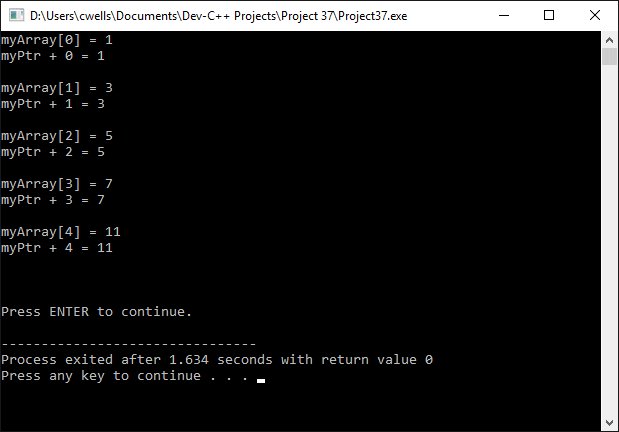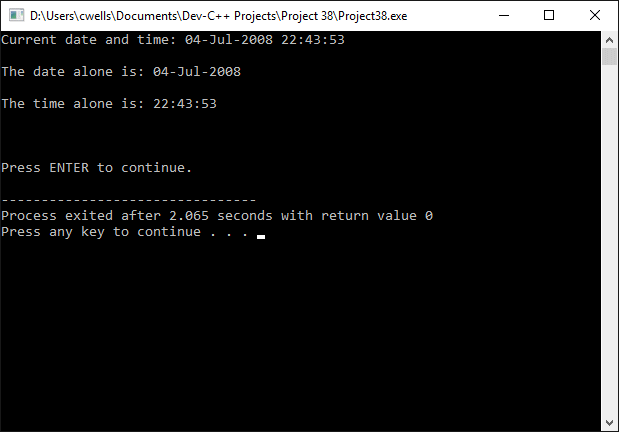Pointers

We have seen that variables can be passed to functions by reference, allowing the function to access the variable using its address in memory, with the possibility of changing the value of the variable itself (as opposed to simply manipulating the value of a copy of the variable). When the memory address of a variable is used in this way, it becomes a special kind of variable in its own right, known as a pointer (because it points to another variable).

The size of a pointer variable depends on the number of bits used by a particular computer system to store memory addresses. The bigger the memory, the more bits are needed. Consider the following declaration:

int *myPtr;

The name of the variable is myPtr. The asterisk (*) tells the compiler that myPtr is a pointer variable, and therefore requires sufficient space in memory to store a memory address. The use of the keyword int indicates that the pointer will hold the address of an integer variable. Initially, the pointer does not have a value, i.e. it is a null pointer.

The address of a variable in memory can be obtained by preceding the variable name with an ampersand (&) in a program statement. The ampersand, when used in this way, is known as the reference (or address of) operator. The following program fragment declares and initialises an integer value (i), and then declares a pointer (myPtr) to type int, which is assigned the address of i.

int i = 10;
int *myPtr = &i;

The process of getting the value pointed to by a pointer variable is called de-referencing the pointer, and is achieved using the dereferencing operator (*), as shown below:

*myPtr = 25;  // this is equivalent to "i = 25;"

This statement assigns a value of 25 to i (not the pointer variable itself).

Pointers and arrays

The type of variable that a pointer variable refers to is important for two reasons. The compiler needs to know what type of variable it is dealing with, and hence the size of the variable, so that it knows how many bytes of memory to set aside for it. The size of the variable is also important, however, when dealing with array variables. If the pointer variable myPtr, for example, points to the first integer variable in an array of 10 integer variables, think about the implications of the following program statement:

myPtr = myPtr + 1;  // (or myPtr++;)

The compiler knows that myPtr is a pointer, and that it points to an integer variable. Assuming that the size of an integer variable on the system in question is four bytes, the above statement assigns an address to myPtr that is four bytes greater than its previous value. Since array variables occupy contiguous memory locations, the pointer myPtr now holds the address of the second integer variable in the array. Incrementing (or decrementing) a pointer value increases (or decreases) the address of the memory location pointed to by a number of bytes equivalent to the size of the variable being pointed at. This is true even if the variable in question is a user-defined type, such as a structure. Incrementing or decrementing a pointer in this way is sometimes referred to as pointer arithmetic, and is often used to traverse an array.

Consider the following:

// Example Program 1

#include <string.h>
#include <iostream>
using namespace std;

int main()
{
int myArray[] = {1, 3, 5, 7, 11};
int *myPtr;
int i;
string s;

myPtr = &myArray;
for(i=0; i<5; i++)
{
cout << "myArray[" << i << "] = " << myArray[i] << "\n";
cout << "myPtr + " << i << " = " << *(myPtr + i) << "\n\n";
}
cout << "\n\nPress ENTER to continue.";
getline( cin, s );
}The output from example program 1

If you type in this program and run it, you will see that the two cout statements inside the for loop produce exactly the same numerical output. The first statement does this by indexing into the array, while the second statement de-references successive pointer values. Note that where we wrote:

myPtr = &myArray;

we could also have writen:

myPtr = myArray;  // the name of the array is a pointer

In C++, as we have already seen, a string can be created using an array of characters terminated with a null character. Because the characters in the string occupy contiguous memory locations, just like the elements in any other type of array, the array can be manipulated using pointers, just as we did with the integer array. The following short program uses pointer arithmetic, together with the memcpy() function, to extract the date and time elements from one string, and insert them into two separate strings.

// Example Program 2

#include <string.h>
#include <iostream>
using namespace std;

int main()
{
char nowStr[] = "Current date and time: 04-Jul-2008 22:43:53";
char dateStr = "";
char timeStr = "";
string s;

memcpy(dateStr, nowStr+23, 11);
memcpy(timeStr, nowStr+35, 9);
cout << nowStr << "\n\n";
cout << "The date alone is: " << dateStr << "\n\n";
cout << "The time alone is: " << timeStr << "\n\n";
cout << "\n\nPress ENTER to continue.";
getline( cin, s );
}The output from example program 2